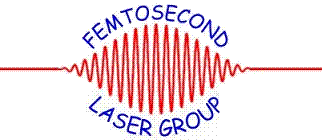## Research Papers in Physics and Astronomy## C.J.G.J. Uiterwaal Publications

#### Date of this Version

January 1995

Published by American Institute of Physics. J. Chem. Physics 102 (2), 8 January 1995. Copyright © 1995 American Institute of Physics. Permission to use.

#### Abstract

Using the photo-electron-product-ion-coincidence method (PEPICO) we have measured state-selective cross sections for the following processes: (A) N2+ (X,A;v)+ H2→N2H+ + H, (B) H2+ (X;v) + N2→N2H+ + H, (C) N2+ (X,A;v)+H2→H2+ + N2, and (D) H2+ (X;v)+N2→N2+ + H2 . The measurements were performed at thermal velocities (Ec.m. ≈ 40 meV).We have found that the charge transfer processes (C) and (D) have cross sections that are at least an order of magnitude smaller than the cross sections for the rearrangement processes (A) and (B). The cross section for reaction (A) with N2+ (A;v) as reactant is found to be (50.2±2.4)% of the cross section for the same reaction with N2+ (X;v) as reactant. The cross section for reaction (B) is found to be independent of the internal energy of the reactant ion. The measured variation of the cross sections as a function of the internal energy of the reacting ion is compared with calculations based on a RRKM type statistical model and an electronic correlation diagram of the (N2–H2)+ system. Excellent agreement is found, indicating complete randomization of internal energy within the collision complex. Absolute cross sections are determined for the rearrangement reactions: for reaction (A) the cross section is 76.1 Å2 starting with N2+ (X;v = 0,1) and 38.05 Å2 starting with N2+ (A;v = 0 –5). For reaction (B) the cross section is 114 Å 2 for H2+ (X;v = 0 –6).

COinS